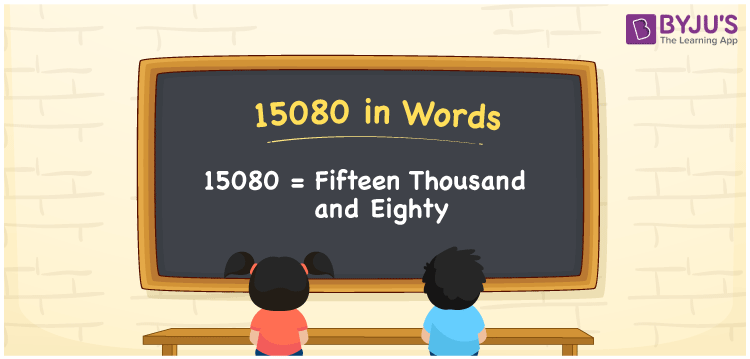# 15080 in Words

Fifteen Thousand and Eighty is the number name for the number 15080. It is a five-digit number. As we know, the number 15080 is a cardinal number since it represents the value equivalent to fifteen thousand and eighty. In this article, let us discuss the number 15080 in words and the process of writing the number name using the place value system in detail.

 15080 in Words: Fifteen Thousand and Eighty Fifteen Thousand and Eighty in Numerical Form: 15080

## 15080 in English Words## How to Write 15080 in Words?

The following table represents the place values of the number 15080:

 Ten-thousands Thousands Hundreds Tens Ones 1 5 0 8 0

The expanded form of 15080 is as follows:

= 1 × Ten thousand + 5 × Thousand + 0 × Hundred + 8 × Ten + 0 × One

= 1 × 10000 + 5 × 1000 + 0 × 100 + 8 × 10 + 0 × 1

= 10000 + 5000 + 80

= 15080

= fifteen thousand and eighty

Hence, 15080 in words is Fifteen Thousand and Eighty.

15080 in words – Fifteen Thousand and Eighty

Is 15080 an odd number? – No

Is 15080 an even number? – Yes

Is 15080 a perfect square number? – No

Is 15080 a perfect cube number? – No

Is 15080 a prime number? – No

Is 15080 a composite number? – Yes

## Frequently Asked Questions on 15080 in Words

Q1

### How to write 15080 in words?

15080 in words is fifteen thousand and eighty.

Q2

### Simplify 15000 + 80, and express it in words.

Simplifying 15000 + 80, we get 15080. Hence, 15080 in words is fifteen thousand and eighty.

Q3

### Is 15080 an odd number?

No, 15080 is not an odd number.

Q4

### How do you write 15080 in Words on a Cheque?

On a cheque, we generally write 15080 in words as Fifteen Thousand and Eighty.
Q5

### Is 15080 a Perfect Square?

No.15080 is not a Perfect Square.